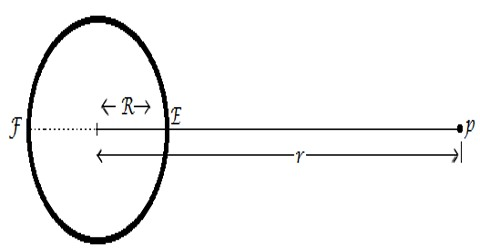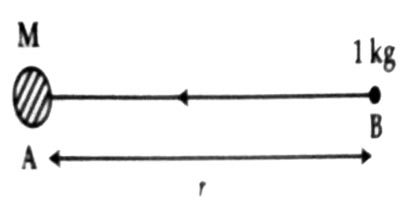# Gravitational Intensity due to a Point Mass

Gravitational Intensity due to a point mass

We distinguish that there are four fundamental forces in nature. The force between two bodies by virtue of its masses is called as gravitational force. Newton proposed a law about the gravitational force between two point masses. Point mass is not the mass of the smaller size rather it is a concept. The mass of any shape and size is called as a point mass, if it is studied from a distance larger than the size of the body.

In order to determine the intensity at point B for a body of mass M at a distance r let us consider that a unit mass is placed at point B [Figure]. In that case the attractive force between M and 1 kg mass will be the intensity of that point.According to Newton’s Law of Gravitation,

Intensity, E = (GM x 1) / r2

so, E = GM / r2

Its direction is along BA.

Special cases:

(i) At all points inside a sphere or a shell intensity is zero.

(ii) Intensity outside a solid sphere or a hollow sphere is found out by considering all the masses concentrated at their centre.

Since the strength and direction of the gravitational attraction is diverse in dissimilar locations, the force experienced by a point mass in this case changes in both magnitude and direction as its location is changed.